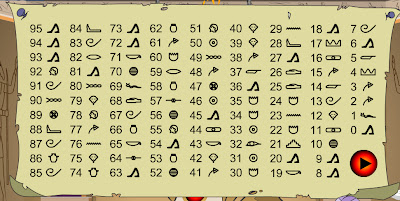## Tuesday, August 10, 2010

### Magic Gopher

Introduction

A friend recently sent me this link to a puzzle.

Click on the link and try out the puzzle before continuing. (If the "music" annoys you it can be turned off at the top right of the screen.)

Spooky!

Before explaining the puzzle I will walk through an example.

The number I picked is 26.

Add 2 and 6 together to get 8.

Subtract 8 from 26 to get 18.

Here is the symbol chart; carefully note the symbol to the right of 18.The gopher displayed that same symbol as shown below:Basic Explanation

Obviously if there was anything "psychic" really going on then the mathematical and symbolic process would not be necessary. It follows that there is some trick in the process.

The basic point is that the maths always results in a number that is a multiple of 9. In the symbol chart all multiples of 9 have the same symbol. Check in the symbol chart above to confirm this. Of cause multiples of 9 are: 9, 18, 27, 36, 45, 54, 63, 72 and 81. The numbers 90 and 99 cannot be produced by the process that the gopher describes. (For example, 99 - 18 = 81). For the explanation of why the process always produces a multiple of 9 see the Matematical Explanation section below.

Every time that you do this puzzle a different symbol chart is used, but always multiples of 9 have the same symbol. Here is another example:So all the gopher has to do is display the symbol for multiples of 9 that are used in the chart that he shows you.

Mathematical Explanation

Some basic maths can explain why the process will always produce a number that is a multiple of 9.

A two digit number can be written as:

10a + b (note 10a is and equivalent way of writing 10 X a)

The gopher asked us to add the two digits together which can be written as (a + b)

He then asked us to take the sum of the digits away from the original number, which can be written as:

10a + b - (a + b)

=10a + b - a - b

Rearranging we get:

10a - a + b - b

The b values cancel (ie b - b = 0) so

10a - a

= 9a

All results of the maths are multiples of 9. In the worked example, the number is 26. In that case a = 2 and b = 6 and 9a = 9 X 2 = 18, a multiple of 9.